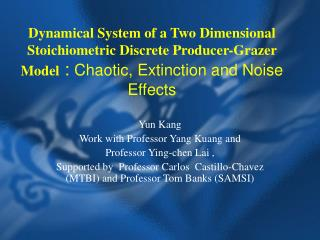DownloadDownload PresentationDynamical System of a Two Dimensional Stoichiometric Discrete Producer-Grazer Model : Chaotic, Extinction and Noise Ef

# Dynamical System of a Two Dimensional Stoichiometric Discrete Producer-Grazer Model : Chaotic, Extinction and Noise Ef

Télécharger la présentation## Dynamical System of a Two Dimensional Stoichiometric Discrete Producer-Grazer Model : Chaotic, Extinction and Noise Ef

- - - - - - - - - - - - - - - - - - - - - - - - - - - E N D - - - - - - - - - - - - - - - - - - - - - - - - - - -
##### Presentation Transcript

1. Dynamical System of a Two Dimensional Stoichiometric Discrete Producer-Grazer Model : Chaotic, Extinction and Noise Effects Yun Kang Work with Professor Yang Kuang and Professor Ying-chen Lai , Supported by Professor Carlos Castillo-Chavez (MTBI) and Professor Tom Banks (SAMSI)

2. Outline of Today’s Talk • Introduce LKE – model, and its corresponding discrete case; • Mathematical Analysis: bifurcation study • Biological Meaning of Bifurcation Diagram; • Chaotic behavior and Extinction of grazer; • Nature of Carry Capacity K and Growth Rate b, and their fluctuation by environments: adding noise • Interesting Phenomenal by adding noise: promote diversity of nature • Conclusion and Future Work

3. Stoichiometry • It refers to patterns of mass balance in chemical conversions of different types of matter, which often have definite compositions

4. most important thing about stoichiometry • we can not combine things in arbitary proportions; e.g., we can’t change the proportion of water and dioxygen produced as a result of making glucose.

5. Energy flowand Element cyclingare two fundamental and unifying principles in ecosystem theory • Using stoichiometric principles, Kuang’s research group construct a two-dimensional Lotka–Volterra type model, we call it LKE-model for short

6. Assumptions of LKE Model • Assumption One: Total mass of phosphorus in the entire system is closed, P (mg P /l) • Assumption Two: Phosphorus to carbon ratio (P:C) in the plant varies, but it never falls below a minimum q (mg P/mg C); the grazer maintains a constant P:C ratio, denoted by (mg P/mg C) • Assumption Three: All phosphorus in the system is divided into two pools: phosphorus in the plant and phosphorus in the grazer.

7. Continuous Model • p is the density of plant (in milligrams of carbon per liter, mg C/l); • g is the density of grazer (mg C/l); • b is the intrinsic growth rate of plant (day−1); • d is the specific loss rate of herbivore that includes metabolic losses (respiration) and death (day−1); • e is a constant production efficiency (yield constant); • K is the plant’s constant carrying capacity that depends on some external factors such as light intensity; • f(p) is the herbivore’s ingestion rate, which may be a Holling type II functional response.

8. Biological Meaning of Minimum Functions • K controls energy flow and (P − y)/q is the carrying capacity of the plant determined by phosphorus availability; • e is the grazer’s yield constant, which measures the conversion rate of ingested plant into its own biomass when the plants are P rich ( ); If the plants are P poor ( ), then the conversion rate suffers a reduction.

9. Continuous Case:b=1.2 and b=2.9

10. Discrete Model From Continuous One • Motivation: Data collect from discrete time, e.g., interval for collecting data is a year. • Biological Meaning of Parameters : Modeling the dynamics of populations with non-overlapping generations is based on appropriate modifications of models with overlapping generations. • Choose

11. Mathematical Analysis • We study the local stability of interior equilibrium E*=(x*,y*)

12. Bifurcation Diagram and Its Biological Meaning • For continuous case: K=1.5

13. Bifurcation Diagrams on Parameter b

14. Bifurcation Diagrams on Parameter b

15. Bifurcation Diagrams on Parameter K

16. Relationship Between K and b: • From these figures, we can see that there is nonlinear relationship between K and b which effect the population of plant and grazer: • For bifurcation of K, increasing the value of b, the diagram of b seems shrink. • For bifurcation of b, increasing the value of K, bifurcation diagram seems move to the left

17. Extinction of Grazer • From bifurcation diagram, we can see that for some range of K and b, grazer goes to extinct. What are the reasons?

18. Basin Boundary For Extinction

19. Global Stability Conjecture • We know that Discrete Rick Model : x(n+1)=x(n)exp{b(1-x(n)/K)} has global stability for b<2, does our system also has this properties More general, if we have u(n+1)=u(n)exp{f(u(n),0} with global stability, then the following discrete system: x(n+1)=x(n)exp{f(x(n),y(n))+g(x(n),y(n))}, of g(x,y) goes to zero as y tending to zero, in which condition has global stability

20. Nature of K and b • K is carrying capacity of plant, and it is usually limited by the intensity of light and space. Since K is easily affected by the environment, it will not be always a constant ; • b is maximum growth rate of plant, it will fluctuates because of environment changing.

21. Adding Noise • Because of the nature of biological meaning of K and b, it makes perfect sense to think these parameters as a random number. • We let K=K0+ w*N(0,1) b=b0+w*N(0,1)

22. Then Most Interesting thing on parameter K : • Prevent extinction of grazer :

23. Time Windows

24. Scaling • Define the degree of existence : R=average population of graze/ average population of plant Then try different amplititute of noise w, then do the log-log scaling, it follows the scaling law.

25. Future Work • We would like to use “snapshot” method to see how noise effects the population of grazer and producer; • Try to different noise, e.g. color noise, to see how the ‘color’ effect the extinction of the grazer;